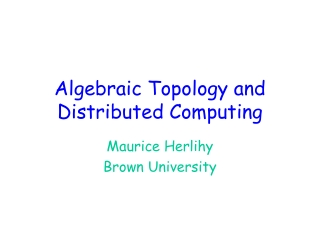DownloadDownload PresentationAlgebraic Topology and Distributed Computing

# Algebraic Topology and Distributed Computing

Download Presentation## Algebraic Topology and Distributed Computing

- - - - - - - - - - - - - - - - - - - - - - - - - - - E N D - - - - - - - - - - - - - - - - - - - - - - - - - - -
##### Presentation Transcript

1. AlgebraicTopology and Distributed Computing Maurice Herlihy Brown University

2. Overview • Focus on applications of algebraic topology to fault-tolerant computing • model • techniques • Joint work with Sergio Rajsbaum, Nir Shavit, Mark Tuttle

3. First Part of Talk • Focus on one problem • Consensus • One model of computation • synchronous message-passing • Motivation: • results not new (those come later) • but illustrate model

4. The Consensus Task Before: private inputs After: agree on one input

5. The Model: Synchronous Message-Passing Round 0 Round 1

7. Summary • Consensus • all processes agree on some input • Model • processes run in lock-step • non-faulty processes broadcast • faulty processes broadcast to subset

8. Road Map • Next: mathematical model • combinatorial topology • no interesting mathematics (yet) • but want to focus on model • and basic approach

9. A Vertex Point in high-dimensional Euclidean Space

10. 0-simplex (vertex) 3-simplex (solid tetrahedron) Simplexes 1-simplex (edge) 2-simplex (solid triangle)

11. Simplicial Complex

12. Simplicial Maps • Vertex-to-vertex map • carrying simplexes to simplexes • induces piece-wise linear map

13. Vertex = Process State Process id (color) 7 Value (input or output)

14. Simplex = Global State

15. Complex = Global States

16. Initial States for Consensus 0 • Processes: blue, red, green. • Independently assign 0 or 1 • Isomorphic to 2-sphere • the input complex 0 1 0 1

17. 0 0 0 1 1 1 Final States for Consensus • Processes agree on 0 or 1 • Two disjoint n-simplexes • the output complex

18. Problem Specification • For each input simplex S • relation D(S) • defines corresponding set of legal outputs • carries input simplex • to output subcomplex

19. 0 0 0 1 1 1 Consensus Specification Simplex of all-zero inputs

20. 0 0 0 1 1 1 Consensus Specification Simplex of all-one inputs

21. 0 0 0 1 1 1 Consensus Specification Mixed-input simplex

22. Protocols • Finite program • starts with input values • fixed number of rounds • halts with decision value

23. Generic Protocol Number of rounds s = empty sequence for (i=0; i<r; i++) { broadcast messages s = s + messages received } return d(s) Decision map

24. Protocol Complex • Each protocol defines a complex • vertex: sequence of messages received • simplex: compatible set of vertexes • Treat as operator on input simplex • Model of computation defines protocol complex properties

25. No messages sent vertexes labeled with input values isomorphic to input simplex Single Input: Round Zero 0 0 0

26. No messages sent vertexes labeled with input values isomorphic to input complex 0 0 1 0 1 Round Zero Protocol Complex

27. 0 0 0 0 0 0 0 0 0 0 0 0 0 0 0 0 0 0 0 0 0 Single Input: Round One red fails green fails no one fails blue fails

28. Protocol Complex: Round One

29. Protocol Complex: Round Two

30. Protocol Complex Evolution zero one two

31. Observation • Decision map • is a simplicial map • vertexes to vertexes, but also • simplexes to simplexes • respects specification relation D

32. Summary d Protocol complex D Input complex Output complex

33. Proof Strategy Find topological “obstruction” to this simplicial map d Protocol complex Output complex

34. Consensus Example Subcomplex of all-zero inputs d Protocol Output must map here

35. Consensus Example Subcomplex of all-one inputs d Protocol Output must map here

36. Contradiction not connected d Protocol Output connected

37. Theorem • In any (n-1)-round protocol complex • the all-zero subcomplex • and the all-one subcomplex • are connected • Corollary: • no (n-1)-round consensus protocol

38. Remarks • Consensus result is • not exactly new [PSL 80] • and doesn’t really need topology • but illustrates basic approach • use topological techniques • to prove non-existence of simplicial map

39. Next Part of Talk • Connectivity of protocol complexes • define notion • Analyze protocol complexes for • message-passing • read/write memory • memory with stronger operations